# Fractions - 6th grade (11y) - math problems

#### Number of problems found: 276

• Write 2Write 791 thousandths as fraction in expanded form.
• Free timeAnita had 2 1/4 hours of free time in the afternoon. She spent 2/3 of her free time playing sports and watched TV for 30 minutes. How much time did she have left to read the book? Express the result in fractions in hours and numbers in minutes.
• Container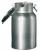How many three-quarters liters jar fill 30 liters container?
• ExpressionIf it is true that ? is:
• Ratio 11Simplify this ratio 10 : 1/4
• A quotientWhat is the quotient of 3/10 divided by 2/4 as a fraction?
• Fraction and a decimalWrite as a fraction and a decimal. One and two plus three and five hundredths
• Pie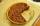Vili ate three pieces of pie . If it piece is 1/8 how much pie did he eat?
• Seeds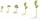Of the 72 wet seeds 7/8 germinated. How many seeds germinate?
• Sum of fractionsWhat is the sum of 2/3+3/5?
• Pounds3 pounds subtract 1/3 of a pound.
• Semicircle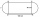Calculate the length of a semicircle with a radius of 6cm.
• Minutes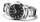Write as fraction in basic form which part of the week is 980 minutes.
• Reciprocal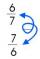Forgiven real numbers, calculate the reciprocal numbers.
• Mixed2improperWrite the mixed number as an improper fraction. 166 2/3
• EquationFind x: x + 1/2 = 1/3
• Minute hand v2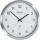In how many minutes describe the minute hand angle 60 degrees?
• Equation 20In given equation: 8/9-4/5=2/9+x, find x
• The quotientThe quotient of g and 55 is the same as 279. What is g?
• Wage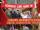Increasing the minimum wage while maintaining the other parameters of the system, unemployment will be:

Do you have an interesting mathematical word problem that you can't solve it? Submit a math problem, and we can try to solve it.

We will send a solution to your e-mail address. Solved examples are also published here. Please enter the e-mail correctly and check whether you don't have a full mailbox.

Please do not submit problems from current active competitions such as Mathematical Olympiad, correspondence seminars etc...

Need help calculate sum, simplify or multiply fractions? Try our fraction calculator.